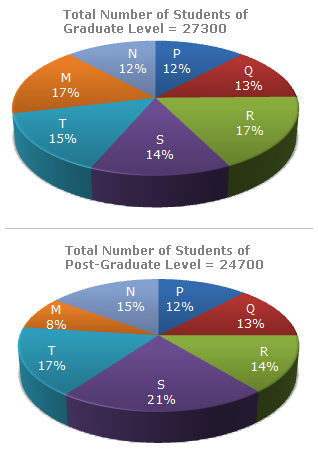# Data Interpretation - Pie Charts - Discussion

The following pie-charts show the distribution of students of graduate and post-graduate levels in seven different institutes in a town.4.

What is the ratio between the number of students studying at post-graduate level from institutes S and the number of students studying at graduate level from institute Q?

 [A]. 13 : 19 [B]. 21 : 13 [C]. 13 : 8 [D]. 19 : 13

Explanation:

 Required ratio = (21% of 24700) = (21 x 24700) = 19 . (13% of 27300) 13 x 27300 13

 Vasavi said: (Feb 16, 2013) Could anyone explain why the % of Graduation for S is taken as 13?, while actually it was given as 14% in the Pie diagram?

 Vijay said: (Mar 5, 2013) How to solve ratio method with calculator and without calculator ?

 Seph said: (Jan 11, 2014) I don't get it. How it arrived with 19:13 as an answer?

 Hima said: (Nov 25, 2014) (21%24700/13%27300) = (19%13).

 Lovely said: (Dec 17, 2014) 21/100*24700:13/100*27300. = 5187:3549 (Divide both sides by 3). = 1729:1183. = (1+7+2+9) : (1+1+8+3). = 19:13.

 Tausif said: (Jan 10, 2015) @Lovely but this is not true for all cases. May please provide logical details.

 Sushil said: (Oct 17, 2015) Please tell how would we calculate ratio for 393/933?

 Liz said: (Dec 6, 2015) Why is it 13% instead of 14% on the pie graph?

 Sanju said: (Nov 13, 2016) Please give me exact calculation without using the calculator.

 Ram said: (May 8, 2018) Graduate students number is 27300. Post graduate student number is 24700.